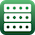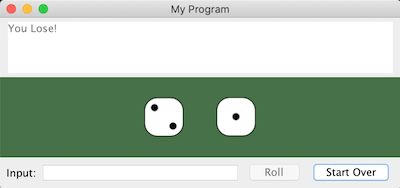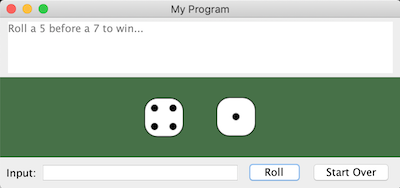Exercises - Booleans and Conditionals

Some of the exercises below provide sample runs to better clarify what the program in question is supposed to do. In these sample runs, text given in green has been typed by the user, while white text has been output by the program. Additionally, the "$" symbol indicates the command prompt, while the "↵" symbol indicates the user has pressed the return key. Exercises identified with a breadboard logo(shown at left) requires the following library: breadboards.jar. 1. Write a class named QuadraticSolver that takes 3 command line arguments representing the (possibly double) values of$a$,$b$, and$c$in the quadratic equation$ax^2 + bx + c = 0$, and then indicates to the user the number of real solutions to that equation and their approximate value(s), as the sample runs below suggest.$ java QuadraticSolver 1 1 1↵
x^2 + x + 1 = 0 has no solutions

$java QuadraticSolver 1 -5 6↵ x^2 - 5x + 6 = 0 has two solutions: 3.0 and 2.0$ java QuadraticSolver 1 0.4 0.04↵
x^2 + 0.4x + 0.04 = 0 has a single solution: -0.2

2. Write a class named BirthdayGuesser that is able to determine the date of the month when someone was born by asking only 5 questions. Each question should ask if the person's birth date is in a given set of integers, as shown below:

Set 1          Set 2          Set 3         Set 4          Set 5
===========    ===========    ===========   ===========    ===========
1  3  5  7     2  3  6  7     4  5  6  7    8  9 10 11    16 17 18 19
9 11 13 15    10 11 14 15    12 13 14 15   12 13 14 15    20 21 22 23
17 19 21 23    18 19 22 23    20 21 22 23   24 25 26 27    24 25 26 27
25 27 29 31    26 27 30 31    28 29 30 31   28 29 30 31    28 29 30 31

The program should keep a running total that starts at 0 and adds $2^{n-1}$ whenever the user indicates his or her birthdate is in set $n$. This total, after all 5 questions are asked, will be the birthdate. A sample run is shown below. Can you explain how this program works and how the initial sets were chosen? (Hint: It has something to do with binary notation.)

$java BirthdayGuesser↵ I will guess the day (i.e., number) of your birthday by asking you only 5 questions about the following sets of numbers! Set 1 Set 2 Set 3 Set 4 Set 5 =========== =========== =========== =========== =========== 1 3 5 7 2 3 6 7 4 5 6 7 8 9 10 11 16 17 18 19 9 11 13 15 10 11 14 15 12 13 14 15 12 13 14 15 20 21 22 23 17 19 21 23 18 19 22 23 20 21 22 23 24 25 26 27 24 25 26 27 25 27 29 31 26 27 30 31 28 29 30 31 28 29 30 31 28 29 30 31 Is the day of your birthday in Set 1 (yes or no)? yes Is the day of your birthday in Set 2 (yes or no)? no Is the day of your birthday in Set 3 (yes or no)? no Is the day of your birthday in Set 4 (yes or no)? no Is the day of your birthday in Set 5 (yes or no)? yes Your birthday is 17 3. Write a class named BmiClassifier that prompts the user for his height (in inches) and weight (in pounds), computes his body mass index (BMI) according to the formula$BMI = h / w^2$where$h$is height in meters and$w$is weight in kilograms. (Recall, there are 0.45359237 kilograms in one pound and 0.0254 meters in one inch.) The program should then print the user's BMI, rounded to two decimal places, and classify the user's weight in accordance with the following, as shown in the sample run. Below 18.5 Underweight 18.5 - 24.9 Normal 25.0 - 29.9 Overweight Above 30.0 Obese$ java BmiClassifier↵
Height (in inches)? 146↵
Weight (in pounds)? 70↵
BMI = 20.95
Normal

4.
import java.util.Scanner;

public class ClassifyChar {

public static void main(String[] args) {

Scanner scanner = new Scanner(System.in);
System.out.println("Enter a character:");

/* NOTE:
* myString.charAt(i) gives the char occupying
* the ith position in the string; the first
* position is given by i = 0
*/
char c = scanner.next().charAt(0);

// we are done collecting input..
scanner.close();

if ((c >= 'a') && (c <= 'z')) {
System.out.println("lowercase letter");
}
else if ((c >= 'A') && (c <= 'Z')) {
System.out.println("uppercase letter");
}
else if ((c >= '0') && (c <= '9')) {
System.out.println("digit");
}
else {
System.out.println("symbol");
}
}
}

5. Write a class named ZodiacSign that takes a positive integer as a command line argument -- one that represents some year -- and prints a message indicating the corresponding year on the Chinese Zodiac. For those not familiar with the Chinese Zodiac, each year is represented by some animal, depending on the remainder upon division of the year by 12 and in accordance with the below table. Sample runs follow.
remainder   animal
=========   =======
0       monkey
1       rooster
2       dog
3       pig
4       rat
5       ox
6       tiger
7       rabbit
8       dragon
9       snake
10       horse
11       sheep

$java ZodiacSign 1900↵ 1900 is the year of the rat$ java ZodiacSign 2017↵
2017 is the year of the rooster

public class ZodiacSign {

public static void main(String[] args) {
int year = Integer.parseInt(args);

String sign = "";

switch (year % 12) {
case 0: sign = "monkey"; break;
case 1: sign = "rooster"; break;
case 2: sign = "dog"; break;
case 3: sign = "pig"; break;
case 4: sign = "rat"; break;
case 5: sign = "ox"; break;
case 6: sign = "tiger"; break;
case 7: sign = "rabbit"; break;
case 8: sign = "dragon"; break;
case 9: sign = "snake"; break;
case 10: sign = "horse"; break;
case 11: sign = "sheep"; break;
}

System.out.println(year + " is the year of the " + sign);
}
}

6. Write a class named IsItALeapYear that takes a positive integer as a command line argument -- one that represents some year -- and prints a message indicating whether or not the supplied year is a leap year. Note, a leap year is one that is divisible by 4 but not by 100, or one that is divisible by 400. Sample runs follow.

$java IsItALeapYear 1971↵ 1971 is not a leap year$ java IsItALeapYear 2016↵
2016 is a leap year

$java IsItALeapYear 1900↵ 1900 is not a leap year$ java IsItALeapYear 2000↵
2000 is a leap year

7.import java.awt.Color;

public class BuffonsNeedle extends Breadboard {

GLine line1;
GLine line2;
GLine needle;
int crossings = 0;
int drops = 0;

public static void main(String[] args) {
new BuffonsNeedle();
}

public BuffonsNeedle() {

// create two horizontal lines spaced 100 pixels apart
this.setSize(500,400);
line1 = new GLine(0,100,500,100);

line2 = new GLine(0,200,500,200);

// create a line to play the role of the needle in our
// experiment.  It will eventually need to stay 100 pixels
// long, but for now we set the start and end points to be
// the same point so that it is essentially invisible
needle = new GLine(0,0,0,0);
needle.setColor(Color.RED);

// set the delay of the breadboard's built-in timer to 100
// milliseconds -- then start the timer
this.getTimer().setDelay(100);
this.getTimer().start();
}

// In the breadboard classes, once their built-in timer has been
// started with a delay of d, the method named "onTimerTick()"
// gets executed repeatedly every d milliseconds
public void onTimerTick() {

// randomly pick an x coordinate between 100 and 400
// (which keeps the point 100 pixels from the left and right edges)
double xStart = 100 + 300 * Math.random();

// randomly pick a y coordinate between 100 and 200
// (which puts this point between the two drawn lines)
double yStart = 100 + 100 * Math.random();

// randomly pick an angle between -pi and +pi
double theta = -Math.PI + 2 * Math.PI * Math.random();

// compute the coordinates of the other end of the 100 pixel
// long needle, as determined by the angle theta
double xEnd = xStart + 100*Math.cos(theta);
double yEnd = yStart - 100*Math.sin(theta);

// update the image of the needle on the canvas
needle.setStartPoint(xStart, yStart);
needle.setEndPoint(xEnd, yEnd);

// update the counts for drops and crossings
drops++;

// a crossing happened if the end point is outside the
// 2 horizontal lines earlier drawn on the canvas
if (yEnd < 100 || yEnd > 200) {
crossings++;
}

// report the new counts and the relevant fraction in the text area
// at the top of the window
String fraction = "2 * drops / crossings = "
+ (2*drops) + "/" + crossings
+ " = " + (2.0 * drops / crossings);

this.getTextArea().setText(fraction);
}
}

8.In the dice game of craps, one rolls two dice and then one of several things happen:

• If you roll a 7 or 11, you win immediately
• If you roll a 2, 3, or 12, you lose immediately
• If you roll any other number (called the "point"), you continue to roll the dice until you see either a 7 or the "point" again). If you see a 7 first, you lose. If you see the "point" first, you win.

Write a class called Craps that extends the Breadboard class to play craps with the user. Once the game is won or lost, the roll button should be disabled until the user clicks the second button on the breadboard, labeled "Start Over" -- at which point the game should be restarted. Some sample screen shots of two games are given below.Winning with an initial roll of 7Losing with an initial roll of 3Establish a point of 5Neither a 7 or the point of 5 was rolled, so roll again.Lose with a point of 5

Hints:

• In the Breadboard class, when the user clicks the first button the method with signature public void onButton1Click() is invoked, if it exists. When the user clicks the second button, the method with signature public void onButton2Click() is similarly invoked.

• One can use the GDieImage class found in the breadboards library to display a die with a given number of dots showing on its top face, as the following code demonstrates:

public class DieTest extends Breadboard {

GDieImage die; // <-- Declare variables here if you want them to
//     be accessible to all methods below,
//     or to a method called more than once.
//     That said, try to put as few variables here
//     as you can -- it will make your code simpler
//     to debug and/or change in the future.

public static void main(String[] args) {
new DieTest();
}

public DieTest()  {
// set the text shown on the two buttons..
this.getButton1().setText("Show");
this.getButton2().setText("Remove");
}

public void onButton1Click() {  // "Show" Button

// creates a die whose face with 3 dots is visible, and
// whose upper left corner is at (100,50)..
die = new GDieImage(3,100,50);

// adds the die to the breadboard window so that it can be seen
}

public void onButton2Click() {  // "Remove" Button

// removes all GObjects from window..
this.removeAll();
}
}

• The following will set the background color of the canvas to a nice "poker-felt green":

final Color POKER_FELT_GREEN = new Color(71,113,72);
this.setBackground(POKER_FELT_GREEN);

• One can enable or disable either button on a TwoButtonBreadboard, as the code below suggests. When a button is disabled, it appears "greyed-out" and does not respond to mouse-clicks.

this.getButton1().setEnabled(false);  // disable the first button
this.getButton2().setEnabled(true);   // enable the second button